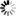Normal view

## An introduction to time series modeling / Andreas Jakobsson

Material type:TextPublication details: Edition: 2. edDescription: 387 s. ill., tab. 23 cmISBN: 9789144108360DDC classification: 519.55 Other classification: Thie | 62M20 | 519.5 | 62M10
Contents:
Introduction -- Stochastic vectors -- Stochastic processes -- Identification and modeling -- Estimation and testing -- Prediction of stochastic processes -- Multivariate processes -- Tracking dynamic systems -- Some useful formulae -- Probability distributions
Summary: Time series analysis concerns the mathematical modeling of time varying phenomena, e.g., ocean waves, water levels in lakes and rivers, demand for electrical power, radar signals, muscular reactions, ECG-signals, or option prices at the stock market. This book gives a comprehensive presentation of stochastic models and methods in time series analysis. The book treats stochastic vectors and both univariate and multivariate stochastic processes, as well as how these can be used to identify suitable models for various forms of observations. Furthermore, different approaches such as least squares, the prediction error method, and maximum likelihood are treated in detail, together with results on the Cramér-Rao lower bound, dictating the theoretically possible estimation accuracy. Residual analysis and prediction of stochastic models are also trated, as well as how one may form time-varying models, including the recursive least squares and the Kalman filter. The book discusses how to implement the various methods using Matlab, and several Matlab functions and data sets are provided with the book
Tags from this library: No tags from this library for this title.Average rating: 0.0 (0 votes)
Item type Current library Call number Status Date due Barcode
Book (loan)
519.5 (Browse shelf (Opens below)) Available 080041489195
##### Browsing Gräsvik shelves, Shelving location: Close shelf browser (Hides shelf browser)
 519.5 Statistisk verktygslåda 2 : multivariat analys / 519.5 Discovering statistics using IBM SPSS statistics : and sex and drugs and rock 'n' roll / 519.5 Tabeller och formler för statistiska beräkningar / 519.5 An introduction to time series modeling / 519.5 Best practices in logistic regression / 519.5 Applied statistics and probability for engineers / 519.5 Statistik med SPSS IBM SPSS Statistics 19 : grunderna /

Innehåller tabeller och diagram

Introduction -- Stochastic vectors -- Stochastic processes -- Identification and modeling -- Estimation and testing -- Prediction of stochastic processes -- Multivariate processes -- Tracking dynamic systems -- Some useful formulae -- Probability distributions

Time series analysis concerns the mathematical modeling of time varying phenomena, e.g., ocean waves, water levels in lakes and rivers, demand for electrical power, radar signals, muscular reactions, ECG-signals, or option prices at the stock market. This book gives a comprehensive presentation of stochastic models and methods in time series analysis. The book treats stochastic vectors and both univariate and multivariate stochastic processes, as well as how these can be used to identify suitable models for various forms of observations. Furthermore, different approaches such as least squares, the prediction error method, and maximum likelihood are treated in detail, together with results on the Cramér-Rao lower bound, dictating the theoretically possible estimation accuracy. Residual analysis and prediction of stochastic models are also trated, as well as how one may form time-varying models, including the recursive least squares and the Kalman filter. The book discusses how to implement the various methods using Matlab, and several Matlab functions and data sets are provided with the book

There are no comments on this title.